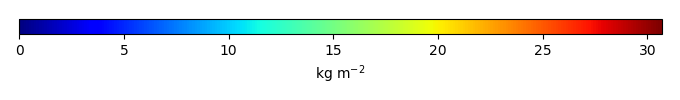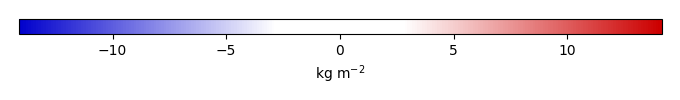# Mean State

Period Mean (original grids) [Pg]
Model Period Mean (intersection) [Pg]
Model Period Mean (complement) [Pg]
Benchmark Period Mean (intersection) [Pg]
Benchmark Period Mean (complement) [Pg]
Bias [kg m-2]
Bias Score 
Spatial Distribution Score 
Overall Score 
Benchmark [-] 26.2
CLM4 [-] 467. 17.5 449. 26.1 0.0878 -0.871 0.62 0.71 0.66
CLM4.5 [-] 453. 17.9 435. 26.1 0.0878 -0.765 0.61 0.82 0.72
CLM5 [-] 487. 15.3 472. 26.1 0.0878 -1.07 0.60 0.79 0.69
Period Mean (original grids) [Pg]
Model Period Mean (intersection) [Pg]
Model Period Mean (complement) [Pg]
Benchmark Period Mean (intersection) [Pg]
Benchmark Period Mean (complement) [Pg]
Bias [kg m-2]
Bias Score 
Spatial Distribution Score 
Overall Score 
Benchmark [-] 0.00338
CLM4 [-] 4.65 0.0142 4.37 0.00338 0.00 0.136 0.57 0.64 0.60
CLM4.5 [-] 8.49 0.0216 8.21 0.00338 0.00 0.274 0.26 0.33 0.29
CLM5 [-] 10.2 0.0260 9.91 0.00338 0.00 0.333 0.27 0.37 0.32
Period Mean (original grids) [Pg]
Model Period Mean (intersection) [Pg]
Model Period Mean (complement) [Pg]
Benchmark Period Mean (intersection) [Pg]
Benchmark Period Mean (complement) [Pg]
Bias [kg m-2]
Bias Score 
Spatial Distribution Score 
Overall Score 
Benchmark [-] 17.5
CLM4 [-] 13.4 13.1 0.201 17.4 0.0333 -0.810 0.66 0.85 0.76
CLM4.5 [-] 14.7 14.3 0.264 17.4 0.0333 -0.452 0.67 0.92 0.80
CLM5 [-] 12.0 11.6 0.234 17.4 0.0333 -1.09 0.65 0.88 0.77
Period Mean (original grids) [Pg]
Model Period Mean (intersection) [Pg]
Model Period Mean (complement) [Pg]
Benchmark Period Mean (intersection) [Pg]
Benchmark Period Mean (complement) [Pg]
Bias [kg m-2]
Bias Score 
Spatial Distribution Score 
Overall Score 
Benchmark [-] 0.222
CLM4 [-] 16.3 0.426 16.0 0.222 0.00 2.45 0.54 0.61 0.57
CLM4.5 [-] 28.1 0.645 27.6 0.222 0.00 5.00 0.39 0.57 0.48
CLM5 [-] 43.6 0.598 43.2 0.222 0.00 4.61 0.41 0.64 0.53
Period Mean (original grids) [Pg]
Model Period Mean (intersection) [Pg]
Model Period Mean (complement) [Pg]
Benchmark Period Mean (intersection) [Pg]
Benchmark Period Mean (complement) [Pg]
Bias [kg m-2]
Bias Score 
Spatial Distribution Score 
Overall Score 
Benchmark [-] 8.47
CLM4 [-] 4.00 4.02 0.0255 8.42 0.0520 -1.04 0.53 0.33 0.43
CLM4.5 [-] 2.99 3.00 0.0350 8.42 0.0520 -1.26 0.48 0.30 0.39
CLM5 [-] 3.04 3.04 0.0355 8.42 0.0520 -1.23 0.50 0.55 0.52

# Temporally integrated period mean

BENCHMARK MEANMODEL MEANBIASBIAS SCORESPATIAL TAYLOR DIAGRAMMODEL COLORS# Spatially integrated regional mean

MODEL COLORS# All Models

BenchmarkCLM4CLM4.5CLM5